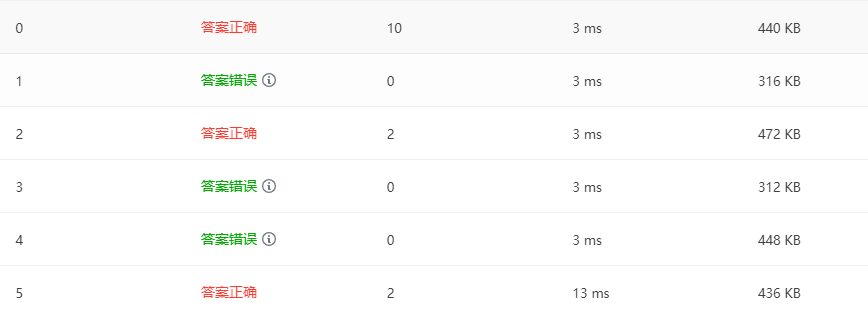2021-11-14 23:22

素数对猜想pta不通过，请问怎么改

#include<iostream>
#include<cmath>
using namespace std;
bool isprime(int a){
for(int i=2;i<=sqrt(a);i++){
if(a%i==0)
return false;
}
return true;
}
int main(){
int N;
cin>>N;
int count=0;
for(int j=3;j<=N;j++){
if(isprime(j)&&isprime(j+2)){
count++;
}
}
cout<<count;
return 0;
}• 写回答
• 好问题 提建议
• 追加酬金
• 关注问题
• 收藏
• 邀请回答

1条回答默认 最新

•ByteSniper 2021-11-14 23:36
已采纳

范围取到N-2就好了 因为是检验j 和 j+2

#include<iostream>
#include<cmath>
using namespace std;
bool isprime(int a){
for(int i=2;i<=sqrt(a);i++){
if(a%i==0)
return false;
}
return true;
}
int main(){
int N;
cin>>N;
int count=0;
for(int j=3;j<=N-2;j++){
if(isprime(j)&&isprime(j+2)){
count++;
}
}
cout<<count;
return 0;
}

评论
解决 无用
打赏 举报 编辑记录